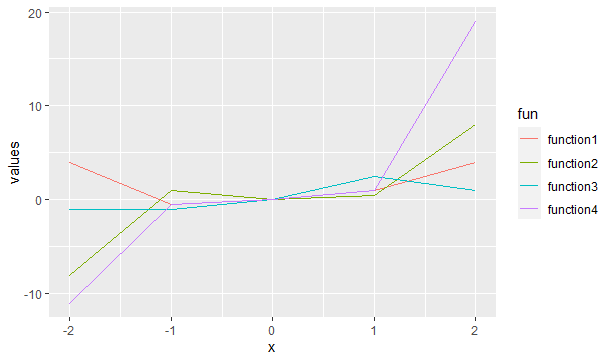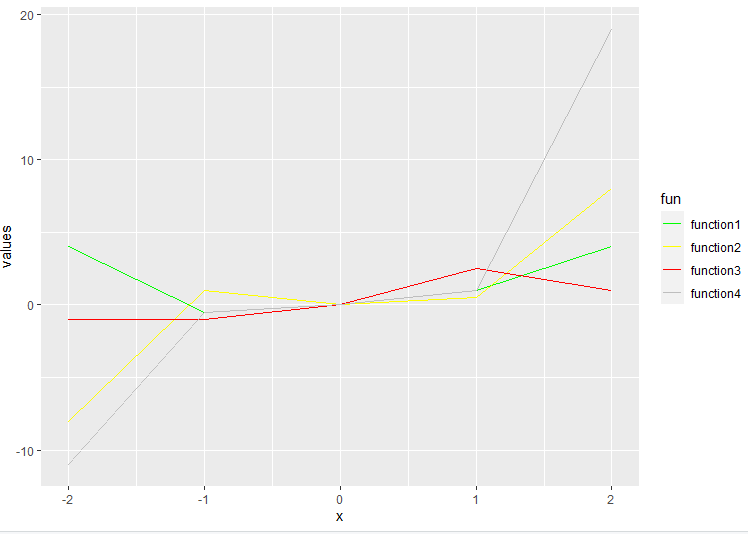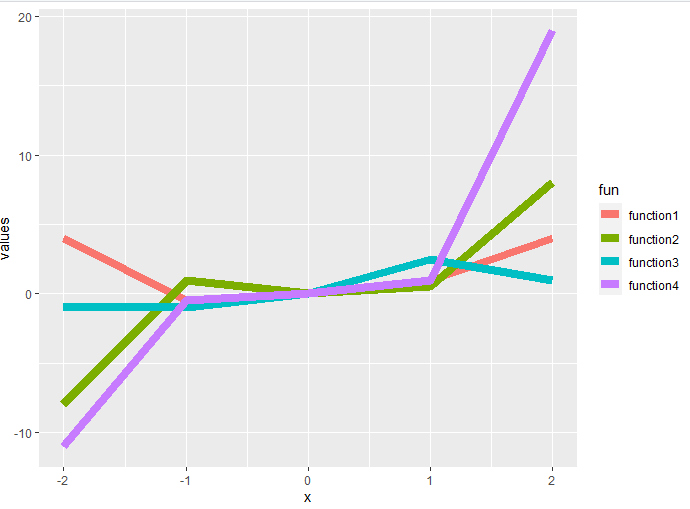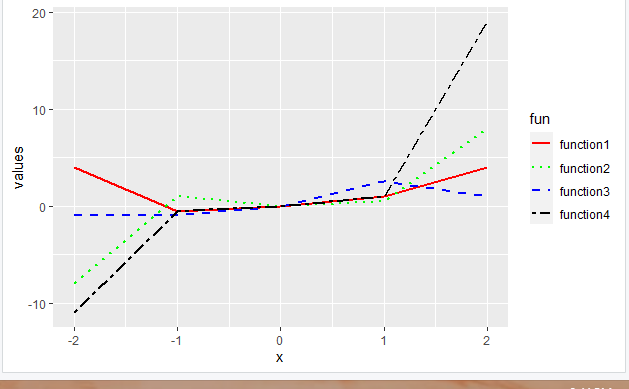Adding Legend to Multiple Line Plots with ggplot in R

• Last Updated : 23 Aug, 2021

In this article, we are going to see how can we add a legend to multiple line plots with ggplot in the R programming language.

For a plot that contains more than one line plot, a legend is created by default if the col attribute is used. All the changes made in the appearance of the line plots will also reflect in the legend.

Syntax: ggplot(df, aes(x, y, col=”name of the column to differentiate on the basis of”))

Let us first visualize how the curve will appear as default.

Example: Default plot

R

 library("ggplot2")  function1<- function(x){x**2}function2<-function(x){x**3}function3<-function(x){x/2}function4<-function(x){2*(x**3)+(x**2)-(x/2)}  df=data.frame(x=-2:2,              values=c(function1(-2:2),                       function2(-2:2),                       function3(-2:2),                       function4(-2:2)),              fun=rep(c("function1","function2","function3","function4")))  ggplot(df,aes(x,values,col=fun))+geom_line()

Output:Various changes made to the lines will appear in the legends as well, let’s see how:

Changing color

To change color by default, the above plot will be produced, now suppose we manually want to add colors to the line, for that any of the given functions- scale_color_manual(), scale_color_brewer(), and scale_color_grey().

Example: changing color of the lines

R

 library("ggplot2")  function1<- function(x){x**2}function2<-function(x){x**3}function3<-function(x){x/2}function4<-function(x){2*(x**3)+(x**2)-(x/2)}  df=data.frame(x=-2:2,              values=c(function1(-2:2),                       function2(-2:2),                       function3(-2:2),                       function4(-2:2)),              fun=rep(c("function1","function2",                        "function3","function4")))  ggplot(df,aes(x,values,col=fun))+geom_line()+  scale_color_manual(values=c("green","yellow","red","grey"))

Output:Changing line thickness

For this, the size attribute is used with a specific value. The changes will be reflected in the legend too.

Example:

R

 library("ggplot2")  function1<- function(x){x**2}function2<-function(x){x**3}function3<-function(x){x/2}function4<-function(x){2*(x**3)+(x**2)-(x/2)}  df=data.frame(x=-2:2,              values=c(function1(-2:2),                       function2(-2:2),                       function3(-2:2),                       function4(-2:2)),              fun=rep(c("function1","function2",                        "function3","function4"))              )  ggplot(df,aes(x,values,col=fun))+geom_line(size=3)

Output:Changing line type

Line type of the plots can be changed using any of the given functions- scale_linetype_manual(), or by default through the use of linetype keyword.

Example:

R

 library("ggplot2")  function1<- function(x){x**2}function2<-function(x){x**3}function3<-function(x){x/2}function4<-function(x){2*(x**3)+(x**2)-(x/2)}  df=data.frame(x=-2:2,              values=c(function1(-2:2),                       function2(-2:2),                       function3(-2:2),                       function4(-2:2)),              fun=rep(c("function1","function2",                        "function3","function4")))  ggplot(df,aes(x,values, group=fun, color=fun, linetype=fun))+geom_line(size=1)+scale_linetype_manual(values = c("solid","dotted","dashed","twodash"))+scale_color_manual(values=c("red","green","blue","black"))

Output:My Personal Notes arrow_drop_up